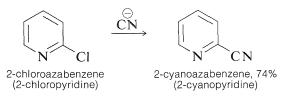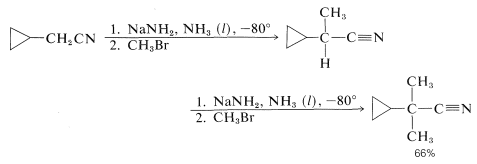# 24.5: Nitriles

$$\newcommand{\vecs}{\overset { \rightharpoonup} {\mathbf{#1}} }$$ $$\newcommand{\vecd}{\overset{-\!-\!\rightharpoonup}{\vphantom{a}\smash {#1}}}$$$$\newcommand{\id}{\mathrm{id}}$$ $$\newcommand{\Span}{\mathrm{span}}$$ $$\newcommand{\kernel}{\mathrm{null}\,}$$ $$\newcommand{\range}{\mathrm{range}\,}$$ $$\newcommand{\RealPart}{\mathrm{Re}}$$ $$\newcommand{\ImaginaryPart}{\mathrm{Im}}$$ $$\newcommand{\Argument}{\mathrm{Arg}}$$ $$\newcommand{\norm}{\| #1 \|}$$ $$\newcommand{\inner}{\langle #1, #2 \rangle}$$ $$\newcommand{\Span}{\mathrm{span}}$$ $$\newcommand{\id}{\mathrm{id}}$$ $$\newcommand{\Span}{\mathrm{span}}$$ $$\newcommand{\kernel}{\mathrm{null}\,}$$ $$\newcommand{\range}{\mathrm{range}\,}$$ $$\newcommand{\RealPart}{\mathrm{Re}}$$ $$\newcommand{\ImaginaryPart}{\mathrm{Im}}$$ $$\newcommand{\Argument}{\mathrm{Arg}}$$ $$\newcommand{\norm}{\| #1 \|}$$ $$\newcommand{\inner}{\langle #1, #2 \rangle}$$ $$\newcommand{\Span}{\mathrm{span}}$$

The carbon-nitrogen triple bond differs considerably from the carbon-carbon triple bond by being stronger ($$212 \: \text{kcal mol}^{-1}$$ vs. $$200 \: \text{kcal mol}^{-1}$$) and much more polar. The degree of polarity of the carbon-nitrogen triple bond is indicate by the high dipole moment $$\left( 4.0 \: \text{D} \right)$$ of the simple nitriles $$\left( \ce{RCN} \right)$$, which corresponds to about $$70\%$$ of the dipole moment expected if one of the bonds of the triple bond were fully ionic. With this knowledge it is not surprising that liquid nitriles have rather high dielectric constants compared to most organic liquids and are reasonably soluble in water. Ethanenitrile, $$\ce{CH_3CN}$$, is in fact a good solvent for both polar and nonpolar solutes (Table 8-5).

Nitriles absorb with variable strength in the infrared in the region $$2000 \: \text{cm}^{-1}$$ to $$2300 \: \text{cm}^{-1}$$, due to stretching vibrations of the carbon-nitrogen triple bond.

The preparation of nitriles by $$S_\text{N}2$$ reactions between alkyl halides and cyanide ion has been mentioned previously (Section 8-7F) and this is the method of choice when the halide is available and reacts satisfactorily. Activated aryl or azaaryl halides similarly give nitriles with cyanide ion:Another practical route to arenecarbonitriles involves the replacement of the diazonium group, $$\ce{-} \overset{\oplus}{\ce{N}} \ce{\equiv N}$$, in arenediazonium ions with cuprous cyanide (Section 23-10B). Other useful syntheses involve cyanohydrin formation (Section 16-4A) and Michael addition to conjugated alkenones (Section 17-5B).

Nitriles also can be obtained by the dehydration of the corresponding amide or aldoxime. This is a widely used synthetic method and numerous dehydrating agents have been found to be effective:The reactions of nitriles include reduction to amines and hydrolysis to acids. Both reactions have been discussed previously (Sections 18-7C and 18-7A).

Hydrogens on the alpha carbons of nitriles are about as acidic as the hydrogens alpha to carbonyl groups; accordingly, it is possible to alkylate the $$\alpha$$ positions of nitriles through successive treatments with a strong base and with an alkyl halide as in the following example: